# Excel Division Operator

## Division Operator

Division uses the `/` symbol in Excel.

How to do division cells:

1. Select a cell and type (`=`)
2. Select a cell
3. Type (`/`)
4. Select another cell
5. Hit enter

You can add more cells to the formula by typing (`/`) between the cells.

Let's have a look at some examples.

## Dividing Manual Entries

Step by step:

1. Type `A1(=)`
2. Type `4/2`
3. Hit enter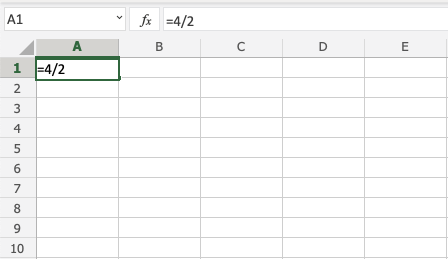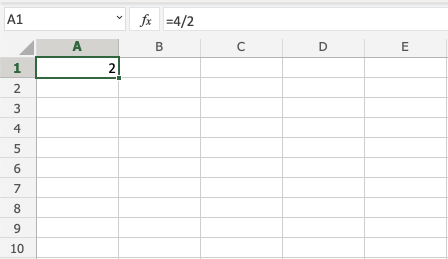## Dividing Two Cells

Let's add some numbers to work with. Type the following values: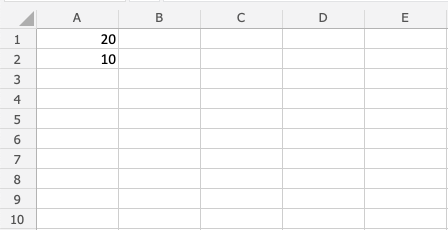Step by step:

1. Type `A3(=)`
2. Select `A1`
3. Type (`/`)
4. Select `A2`
5. Hit enter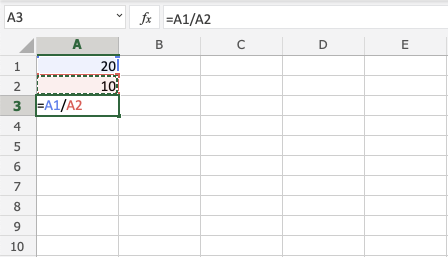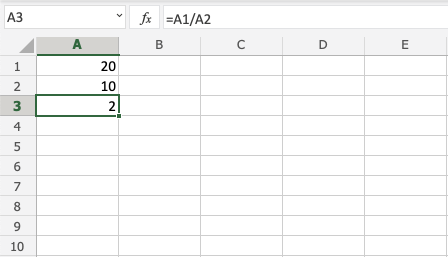## Dividing Using Absolute Reference

You can lock a cell and divide it with other cells.

How to do it, step by step:

1. Select a cell and type (`=`)
2. Select the dividend
3. Type (`/`)
4. Select the divisor lock and add two dollar signs (`\$`) before the column and row
5. Hit enter
6. Fill the range

Note: Dividend is the number being divided by the divisor.

Let's have a look at an example where we divide `B(5)` with the range `A1:A10` using absolute reference and fill function.

Type the values: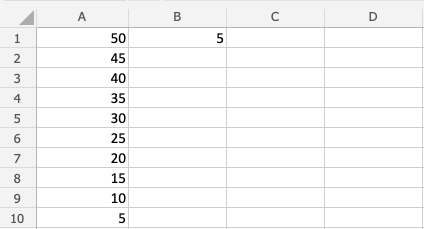Step by step:

1. Type `C1(=)`
2. Select `A1`
3. Type (`/`)
4. Select `B1` type dollar sign before column and row `\$B\$1`
5. Hit enter
6. Fill `C1:C10`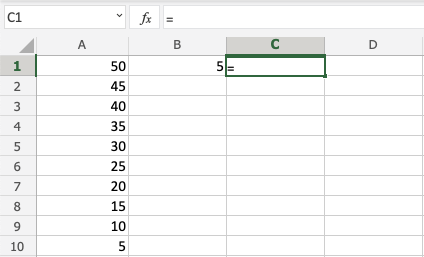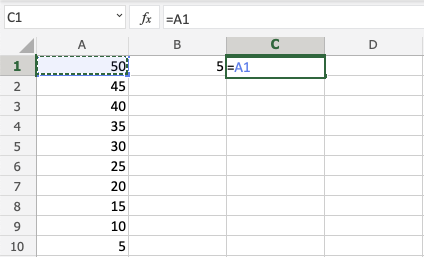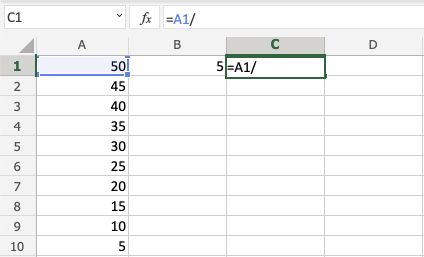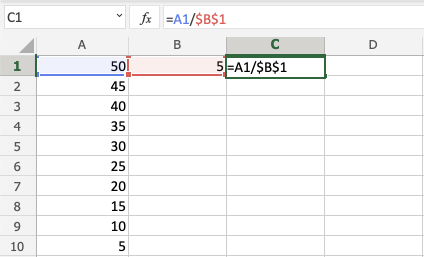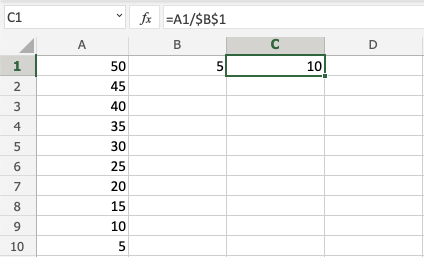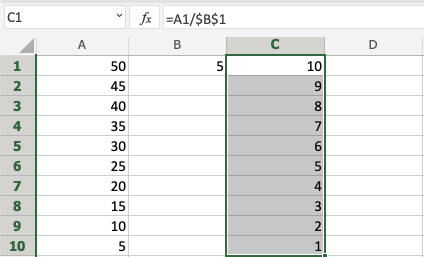Goob job! You have successfully used absolute reference to divide `B1(5)` with the range `A1:A10`.